## Example Questions

### Example Question #98 : Trigonometry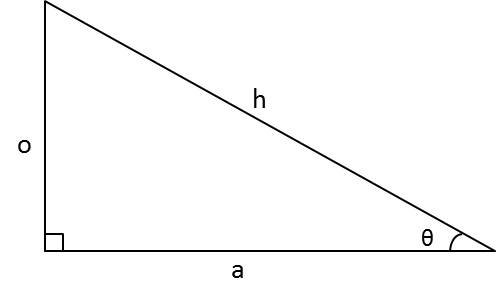In the above triangle,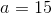and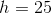. Find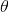.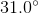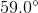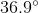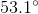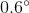Explanation:

With right triangles, we can use SOH CAH TOA to solve for unknown side lengths and angles. For this problem, we are given the adjacent and hypotenuse sides of the triangle with relation to the angle. With this information, we can use the cosine function to find the angle.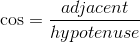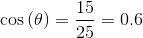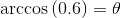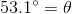### Example Question #1 : How To Find An Angle With CosineFor the above triangle,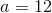and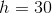. Find.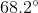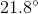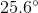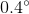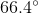Explanation:

With right triangles, we can use SOH CAH TOA to solve for unknown side lengths and angles. For this problem, we are given the adjacent and hypotenuse sides of the triangle with relation to the angle. With this information, we can use the cosine function to find the angle.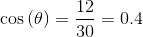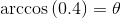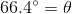### Example Question #100 : TrigonometryFor the above triangle,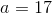and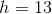. Find.

This triangle cannot exist.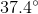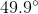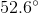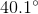This triangle cannot exist.

Explanation:

With right triangles, we can use SOH CAH TOA to solve for unknown side lengths and angles. For this problem, we are given the adjacent and hypotenuse sides of the triangle with relation to the angle. However, if we plug the given values into the formula for cosine, we get: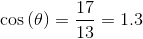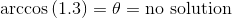This problem does not have a solution. The sides of a right triangle must be shorter than the hypotenuse. A triangle with a side longer than the hypotenuse cannot exist. Similarly, the domain of the arccos function is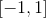. It is not defined at 1.3.

### Example Question #101 : Trigonometry

A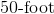rope is thrown down from a building to the ground and tied up at a distance of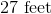from the base of the building. What is the angle measure between the rope and the ground? Round to the nearest hundredth of a degree.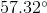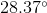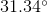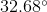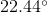Explanation:

You can draw your scenario using the following right triangle: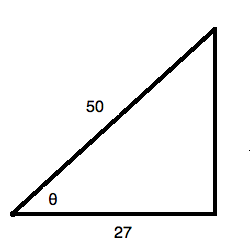Recall that the cosine of an angle is equal to the ratio of the adjacent side to the hypotenuse of the triangle. You can solve for the angle by using an inverse cosine function: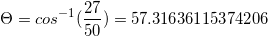or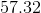degrees.

### Example Question #102 : TrigonometryWhat is the value of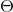in the right triangle above? Round to the nearest hundredth of a degree.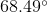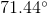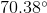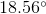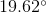Explanation:

Recall that the cosine of an angle is equal to the ratio of the adjacent side to the hypotenuse of the triangle. You can solve for the angle by using an inverse cosine function: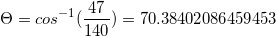or.

### Example Question #103 : Trigonometry

A support beam (buttress) lies against a building under construction. If the beam isfeet long and strikes the building at a pointfeet up the wall, what angle does the beam strike the building at? Round to the nearest degree.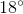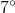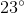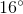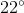Explanation:

Our answer lies in inverse functions. If the buttress isfeet long and isfeet up the ladder at the desired angle, then: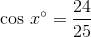Thus, using inverse functions we can say that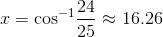Thus, our buttress strikes the buliding at approximately aangle.

### Example Question #104 : Trigonometry

A stone monument stands as a tourist attraction. A tourist wants to catch the sun at just the right angle to "sit" on top of the pillar. The tourist lies down on the groundmeters away from the monument, points the camera at the top of the monument, and the camera's display reads "DISTANCE --METERS". To the nearest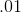degree, what angle is the sun at relative to the horizon?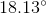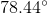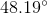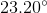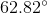Explanation:

Our answer lies in inverse functions. If the monument ismeters away and the camera ismeters from the monument's top at the desired angle, then: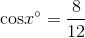Thus, using inverse functions we can say that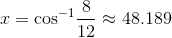Thus, our buttress strikes the buliding at approximately aangle.